# Introduction¶

We begin by looking at a data set to make things concrete.

In :
import pandas as pd

In :
import matplotlib.pyplot as plt

fig,axes=plt.subplots(nrows=2,ncols=2,figsize=(8,8))
axes[0,0].scatter(df['Cigarettes'],df['Lung'])
axes[0,0].set_title('Lung')
#plt.xlabel('Cigarettes Sold per Capita')
axes[0,0].set_xticks([0,1500,3000,4500])
axes[0,0].axis([0,4500,0,30])
axes[0,0].set_yticks(range(0,40,10))

#plt.xlabel('Cigarettes Sold per Capita')
axes[1,0].set_xticks([0,1500,3000,4500])
axes[1,0].set_yticks(range(0,40,10))

axes[0,1].scatter(df['Cigarettes'],df['Leukemia'],color='green')
axes[0,1].set_title('Leukemia')
#plt.xlabel('Cigarettes Sold per Capita')
axes[0,1].set_xticks([0,1500,3000,4500])
axes[0,1].set_yticks(range(0,40,10))

axes[1,1].scatter(df['Cigarettes'],df['Kidney'],color='red')
axes[1,1].set_title('Kidney')
#plt.xlabel('Cigarettes Sold per Capita')
axes[1,1].set_xticks([0,1500,3000,4500])
axes[1,1].set_yticks(range(0,40,10))

fig.suptitle("Deaths from Cancer per 100K Population\n vs \n Cigarette sales per capita")

plt.show()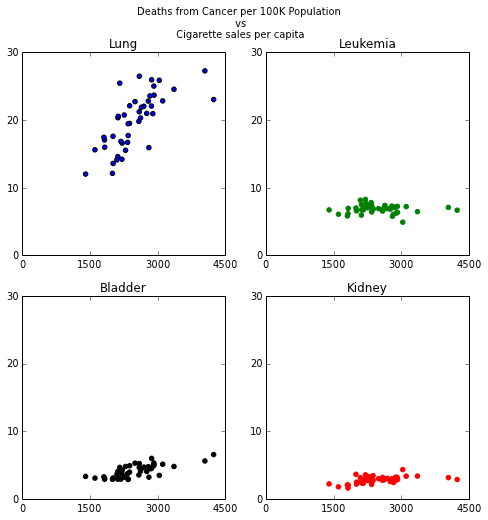In :
fig,axes=plt.subplots(nrows=1,ncols=1,figsize=(10,6))
axes.scatter(df['Cigarettes'],df['Lung'])
axes.set_title('Lung Cancer per 100K Population\n vs \n Cigarette Sales per Capita')
#plt.xlabel('Cigarettes Sold per Capita')
axes.set_xticks([0,1500,3000,4500])
axes.axis([0,4500,0,30])
axes.set_yticks(range(0,40,10))
plt.show()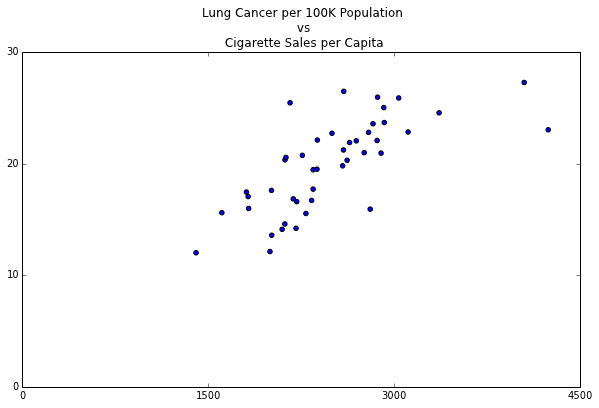There are 44 data points in the set (each corresponds to a state.)

In :
df[['Cigarettes','Lung']][0:10]

Out:
Cigarettes Lung
0 1820 17.05
1 3034 25.88
2 2582 19.80
3 1824 15.98
4 2860 22.07
5 3110 22.83
6 3360 24.55
7 4046 27.27
8 2827 23.57
9 2010 13.58

10 rows × 2 columns

The basic problem of simple linear regression is to find the slope $m$ and intercept $b$ so that the equation of the line $$y=mx+b$$ is the best fit to the data above.

To be specific, we look at the function $$E(m,b)=\sum_{i=1}^{N} (y_i-mx_i-b)^2$$ and we want to find $m$ and $b$ so that this is as small as possible. (For our example, $N=44$.)

# Calculus¶

Although the equation for $E$ looks complicated, it is really a function of two variables and so we can use calculus to find the minimum value. We compute: $$\frac{\partial E}{\partial m}=\sum_{i=1}^{N} 2(y_i-mx_i-b)x_i$$ and $$\frac{\partial E}{\partial b}=\sum_{i=1}^{N} 2(y_i-mx_i-b).$$

Remembering that the variables are $m$ and $b$, set these two equations to zero and find the following two equations: $$S_{yx}=S_{xx}m+S_{x}b$$ and $$S{y}=S{x}m+Nb$$ where I've written $S_{x}=\sum_{i=1}^{N} x_i$, $S_{xy}=\sum_{i=1}^{N} x_iy_i$, and so on.

In :
Sx=sum(df['Cigarettes'])
Sy=sum(df['Lung'])
Sxy=sum([df['Cigarettes'][i]*df['Lung'][i] for i in range(len(df['Cigarettes']))])
Sxx=sum([df['Cigarettes'][i]*df['Cigarettes'][i] for i in range(len(df['Cigarettes']))])
N=len(df['Cigarettes'])
print 'N=',N,'Sx=',Sx,'Sy=',Sy,'Sxx=',Sxx,'Sxy=',Sxy

N= 44 Sx= 109622 Sy= 874.74 Sxx= 286469700 Sxy= 2248867.14


These are two equations in two unknowns that you can solve "by hand" or, for the general solution, you can use Cramer's rule. Let $$M=(NS{yx}-S{x}S_{y})$$ $$B=(S{xx}S_y-S{yx}S_x)$$ $$D=(S{xx}N-S{x}^2)$$ Then $$m=M/D$$ and $$b=B/D.$$

In :
M=N*Sxy-Sx*Sy
B=Sxx*Sy-Sxy*Sx
D=N*Sxx-Sx*Sx
m=M/D
b=B/D
print 'm=',m,'b=',b

m= 0.00520586968046 b= 6.91050349746

In :
fig,axes=plt.subplots(nrows=1,ncols=1,figsize=(10,6))
axes.scatter(df['Cigarettes'],df['Lung'])
axes.plot(np.arange(0,4500,1),m*np.arange(0,4500,1)+b*np.ones(len(np.arange(0,4500,1))),color="red",label='Regression Line')
axes.set_title('Lung Cancer per 100K Population\n vs \n Cigarette Sales per Capita')
#plt.xlabel('Cigarettes Sold per Capita')
axes.set_xticks([0,1500,3000,4500])
axes.axis([0,4500,0,30])
axes.set_yticks(range(0,40,10))
plt.legend()
plt.show()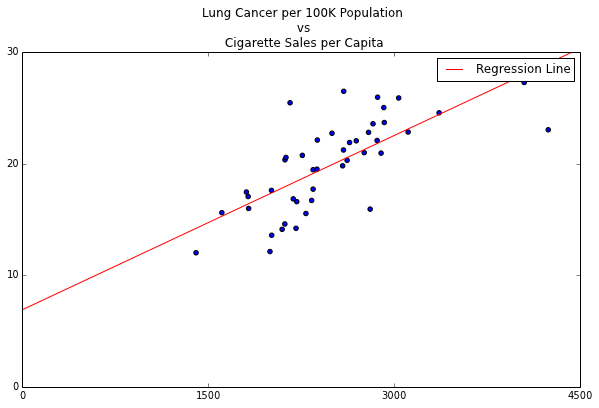# Linear Algebra¶

Let's look at the problem from a different point of view. Let's consider three column vectors:

• $Y=\left[\begin{matrix} y_1 \cr y_2 \cr \vdots \cr y_N\end{matrix}\right]$
• $X=\left[\begin{matrix} x_1 \cr x_2 \cr \vdots \cr x_N\end{matrix}\right]$
• $E=\left[\begin{matrix} 1 \cr 1 \cr \vdots \cr 1 \end{matrix}\right]$

If things were "perfect", meaning that the points all belonged to the line $y=mx+b$, we would have $$Y=mX+bE.$$

But, of course, we don't. In linear algebra terms, the vector $Y$ does not lie in the plane spanned by $X$ and $E$ in $\mathbb{R}^{44}$

So let's try to find the point in the plane spanned by $E$ and $X$ which is closest to $Y$.

In :
from mpl_toolkits.mplot3d import Axes3D
#plt.subplots(nrows=1,ncols=1,figsize=(6,10))
fig=plt.figure(figsize=(10,10))
X = np.arange(-2, 2, 0.25)
Y = np.arange(-2, 2, 0.25)
X, Y = np.meshgrid(X, Y)
Z=2*Y-X
t=np.arange(-1,0,.1)
ax.plot_wireframe(X,Y,Z)
ax.plot(t,-2*t,6+t,color="red")
ax.scatter([0,-1],[0,2],[6,5],color="red")
ax.set_title("Closest point lying in a given plane from a point outside the plane")
plt.show()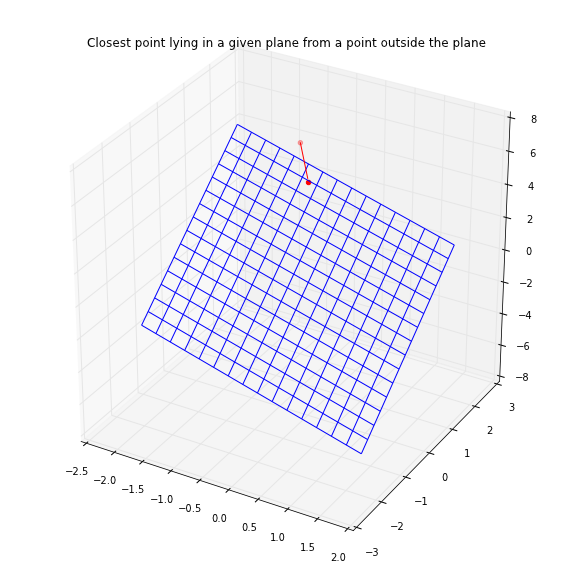We can use linear algebra to solve the problem of finding the point in the plane $P$ spanned by $X$ and $E$ that is closest to $Y$. Essentially, we need to take the perpendicular projection of the vector $Y$ into the plane spanned by $X$ and $E$. For that, we can construct an orthonormal basis for the plane $P$ by using the Gram-Schmidt process on the two vectors $E$ and $X$.

First, we normalize $E$ to obtain our first unit vector $\mathbf{e}=E/\sqrt{N}$, since $E\cdot E=N$.

Next, we subtract the projection of $X$ onto the $E$ direction and normalize. Let $x=X-(X\cdot \mathbf{e})\mathbf{e}$ and $$\mathbf{x}=\frac{x}{\sqrt{x\cdot x}}$$

Then the point we are interested in is $$\mathbf{y}=(Y\cdot\mathbf{x})\mathbf{x}+(Y\cdot\mathbf{e})\mathbf{e}$$

This point lies in the plane $P$, and $Y-\mathbf{y}$ is perpendicular to both $E$ and $X$.

Let's make a few observations about this. First of all, $(Y\cdot\mathbf{e})\mathbf{e}=\overline{y}E$ and $(X\cdot \mathbf{e})\mathbf{e}=\overline{x}E$ where $\overline{x}$ and $\overline{y}$ are the averages (means) of the $x$ and $y$ values respetively.

We can arrange for our data to have $\overline{x}=0$ and $\overline{y}=0$ by subtracting $\overline{x}$ and $\overline{y}$ each of the points. This amounts to a coordinate change that moves the origin of our coordinate system to $(\overline{x},\overline{y})$.

In these coordinates, we get a huge simplification. If both means are zero, we get $$\mathbf{x}=\frac{X}{\sqrt{X\cdot X}}$$ and $$\mathbf{y}=\frac{Y\cdot X}{X\cdot X}X$$ In other words, in these coordinates, $b=0$ and $m=\frac{Y\cdot X}{X\cdot X}$.

In :
Xnorm=df['Cigarettes']-df['Cigarettes'].mean()
Ynorm=df['Lung']-df['Lung'].mean()
plt.scatter(Xnorm,Ynorm)
m=Xnorm.dot(Ynorm)/Xnorm.dot(Xnorm)
plt.plot(Xnorm, m*Xnorm)
plt.show()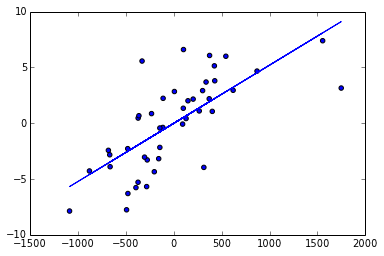# Statistics¶

What accounts for the variation of the points around the "true value"? Statisticians approach this through the idea of a statistical model. Let's leave the lung cancer data behind for the moment, and imagine an abstract problem. Suppose that we make measurements of a dependent variable $Y$ that is related to an independent variable $X$ by a linear equation. Suppose, however, that the $Y$ values include a certain amount of random error $\epsilon$, so that $$Y=mX+b+\epsilon.$$

We will assume that the error term $\epsilon$ is a normally distributed error, with mean zero, variance $\sigma$, and that the errors we obtain from separate measurements of $Y$ are independent of one another.

In :
from scipy.stats import norm
X=np.arange(-5,5,.1)
plt.plot(X,norm.pdf(X,scale=.7,loc=0),color='red',label='v=.5')
plt.plot(X,norm.pdf(X,scale=1),color='blue',label='v=1')
plt.plot(X,norm.pdf(X,scale=1.5),color='orange',label='v=2.25')

plt.title('Normal Distributions')
plt.legend()
plt.show()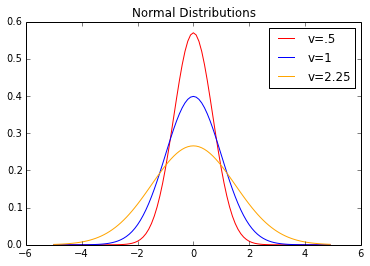Suppose for the sake of concreteness that the $X$ values are 0.0,0.1,...,9.9 and that $Y=X+1+\epsilon$ where $\epsilon$ has variance $1$. Different measurements create different scatter plots.

In :
def MB(X,Y):
Sxx=sum([X[i]*X[i] for i in range(len(X))])
Sxy=sum([X[i]*Y[i] for i in range(len(X))])
Sx=sum([X[i] for i in range(len(X))])
Sy=sum([Y[i] for i in range(len(Y))])
N=len(X)
M=N*Sxy-Sx*Sy
B=Sxx*Sy-Sxy*Sx
D=N*Sxx-Sx*Sx
m=M/D
b=B/D
return m,b

X=np.arange(0,10,.1)
fig,ax=plt.subplots(nrows=2,ncols=2,figsize=(6,6))
epsilon=norm.rvs(size=100,scale=2,loc=0)
Y0=X+np.ones(len(X))+epsilon
epsilon=norm.rvs(size=100,scale=2,loc=0)
Y1=X+np.ones(len(X))+epsilon
epsilon=norm.rvs(size=100,scale=2,loc=0)
Y2=X+np.ones(len(X))+epsilon
epsilon=norm.rvs(size=100,scale=2,loc=0)
Y3=X+np.ones(len(X))+epsilon
for i in [0,1]:
for j in [0,1]:
ax[i,j].set_xticks(range(0,11,2))
ax[i,j].set_yticks(range(-5,17,2))
ax[0,0].scatter(X,Y0,color='blue')
ax[1,0].scatter(X,Y1,color='red')
ax[0,1].scatter(X,Y2,color='green')
ax[1,1].scatter(X,Y3,color='black')
m0,b0=MB(X,Y0)

m1,b1=MB(X,Y1)

m2,b2=MB(X,Y2)
m3,b3=MB(X,Y3)
ax[0,0].plot(X,m0*X+b0,color="blue")
ax[1,0].plot(X,m1*X+b1,color="red")
ax[0,1].plot(X,m2*X+b2,color="green")
ax[1,1].plot(X,m3*X+b3,color="black")
plt.show()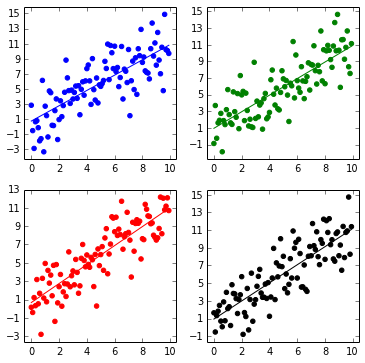We can superimpose these different lines and you can see that they are slightly different -- with varying slope and intercept -- which come from different snapshots of the underlying random data.

In :
plt.plot(X,m0*X+b0,color="blue")
plt.plot(X,m1*X+b1,color="red")
plt.plot(X,m2*X+b2,color="green")
plt.plot(X,m3*X+b3,color="black")
plt.show()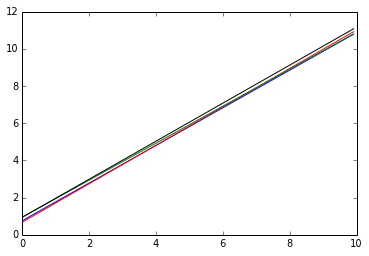Suppose we draw 5000 samples of 100 Y-values each, where each of the 100 $Y$ values from a given sample satisfy $Y=X+1+\epsilon$. For each of the 5000 samples, we calculate the slope and intercept for those $100$ $Y$-values. The picture below shows that these slope/intercept pairs lie in an ellipse centered at $(1,1)$.

In :
Y=X+np.ones(len(X))
M,B=[],[]
for i in range(5000):
Yn=Y+norm.rvs(size=100,loc=0,scale=2)
m,b=MB(X,Yn)
M.append(m)
B.append(b)
plt.title("Slope/Intercept Pairs for Different Samples")
plt.xlabel("Slope")
plt.ylabel("Intercept")
plt.scatter(B,M,s=.3,alpha=.3,color='blue')
plt.show()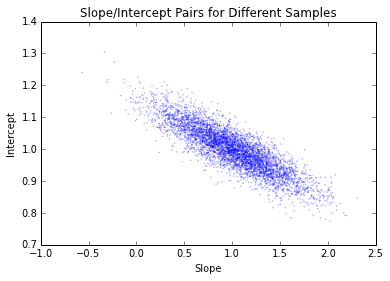To see where this ellipse comes from, let's recall the orthonormal vectors $e$ and $x$ introduced earlier. We saw that the predicted $Y$ value corresponds to the orthogonal projection $\hat{Y}=(Y\cdot e)e+(Y\cdot x)x$. The points $((Y\cdot e),(Y\cdot x))$ and $(Y\cdot x)$ are distributed around $((X+1)\cdot e),((X+1)\cdot x))$ -- basically we are looking at the random vectors $\epsilon$ projected orthogonally onto the $e,x$ plane. Since the $\epsilon$ fall in a sphere around zero, the projection is a circle.

In :
def P(X,Y):
N=len(X)
Xbar=sum(X)/N
Sx=sum((X-Xbar*np.ones(N))**2)
A=sum(Y)/sqrt(N)
Z=(X-Xbar*np.ones(N))/sqrt(Sx)
B=sum([Z[i]*Y[i] for i in range(N)])
return A,B

A=[]
B=[]
a0,b0=P(X,X+1)
for i in range(5000):
Yn=Y+norm.rvs(size=100,loc=0,scale=2)
a,b=P(X,Yn)
A.append(a-a0)
B.append(b-b0)

fig,axes=plt.subplots(nrows=1,ncols=2,figsize=(10,10))
axes.set_title("Orthogonal\n Projection of Residuals")
axes.axis([-10,10,-10,10])
axes.set_aspect('equal')
#plt.gca().set_aspect('equal')
axes.scatter(A,B,s=.3,alpha=.3,color='blue')

axes.hist(A,normed='True')
axes.hist(B,normed='True')
axes.plot(np.arange(-10,10,.1),norm.pdf(np.arange(-10,10,.1),scale=2),color='black')
axes.set_title("Distribution of Residuals")

plt.show()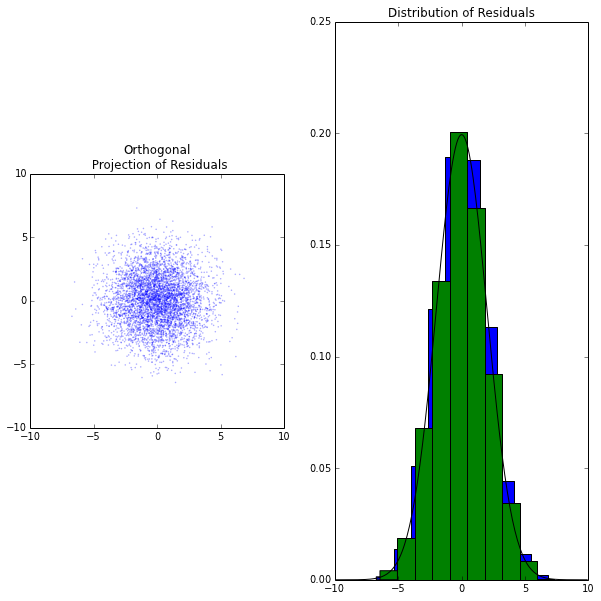We can make a change of variables from the $x$,$e$ coordinate system to the $X$, $E$ coordinates; the coefficients of the $\hat{Y}$ vector in these coordinates are $m$ and $b$. The circular cloud of points becomes this ellipse. The ellipses correspond to the circles where about 68\% of the points lie, 95\%; and 99.7\%.

In :
#plt.xlabel("Slope")
#plt.ylabel("Intercept")
#axes.set_xticks(np.arange(-.5,.5,.1))
fig=figure(num=1,figsize=(10,10))

axes=fig.gca()

A=np.array(A)
B=np.array(B)
axes.set_title('Projection of Residuals in Slope,Intercept')
axes.axis([-2,2,-.3,.3])
axes.set_xticks(np.arange(-2,3,1))
N=len(X)
Xbar=sum(X)/N
Sx=sum((X-Xbar*np.ones(N))**2)
R=Sx+N*Xbar**2
S=2*N*Xbar
T=N
u=np.arange(-3,4,.01)
v=np.arange(-3,4,.01)
U,V=np.meshgrid(u,v)
axes.contour(U,V,T*U**2+S*U*V+R*V**2,levels=[4,16,36],colors=['black','black','black'])
axes.scatter(1/sqrt(N)*A-Xbar/sqrt(Sx)*B,1/sqrt(Sx)*B,s=.5,alpha=.3,color='red')
plt.show()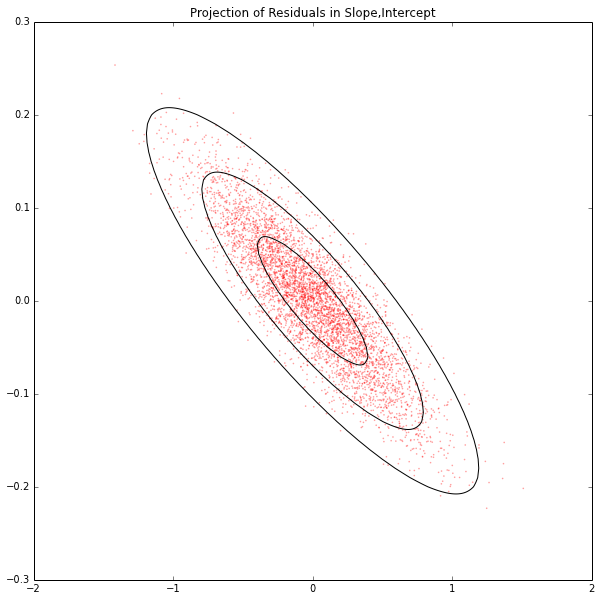In :


In :
In :
In :


In [ ]: